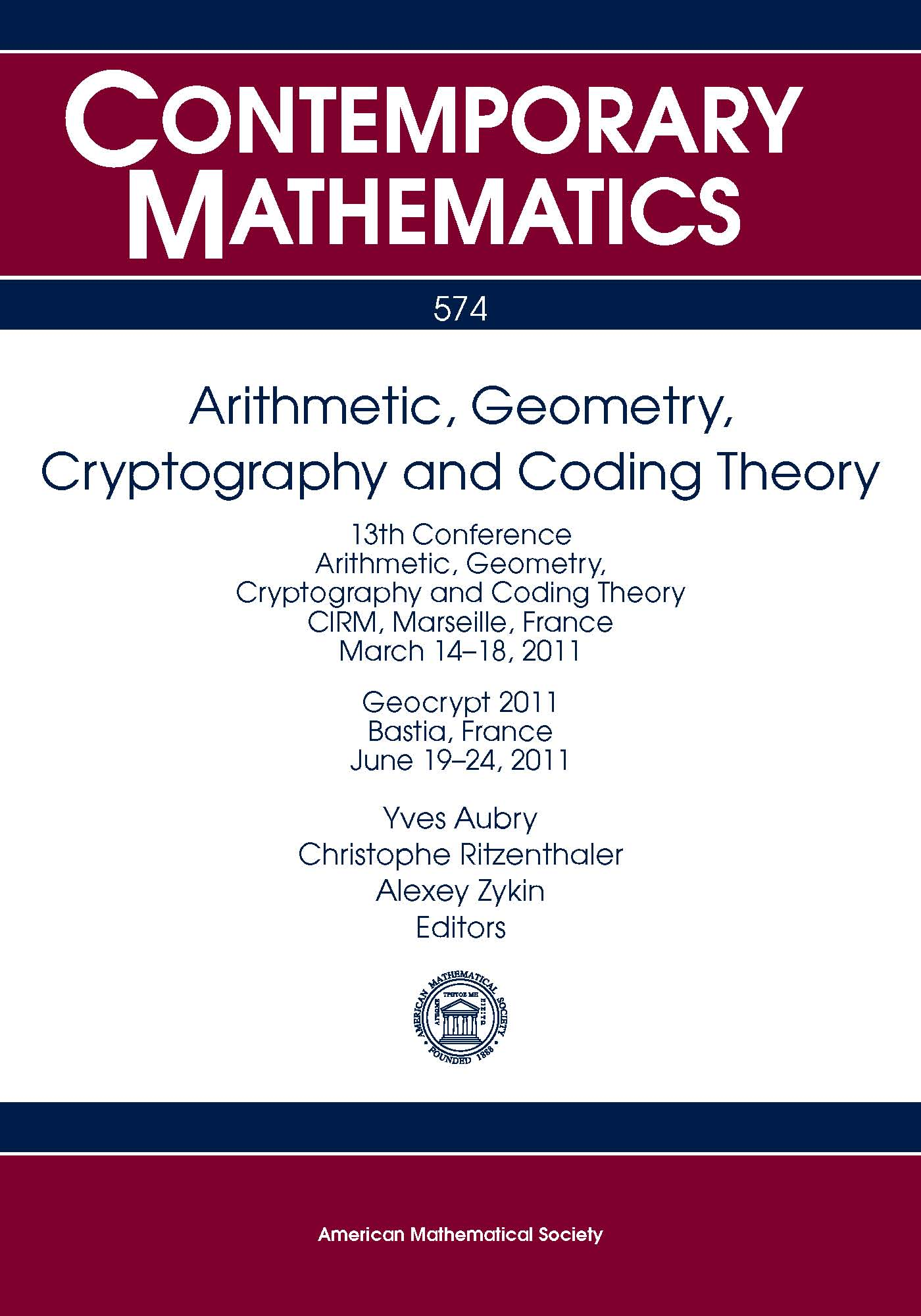• A
• A
• A
• ABC
• ABC
• ABC
• А
• А
• А
• А
• А
Regular version of the site

## Arithmetic, Geometry, Cryptography and Coding Theory (2012)

Iss. 574. Providence: American Mathematical Society, 2012.
Under the general editorship: A. I. Zykin, Y. Aubry, C. Ritzenthaler.

The volume is to contain the proceedings of the 13th conference AGCT as well as the proceedings of the conference Geocrypt. The conferences focus on various aspects of arithmetic and algebraic geometry, number theory, coding theory and cryptography. The main topics discussed at conferences include the theory of curves over finite fields, theory of abelian varieties both over global and finite fields, theory of zeta-functions and L-functions, asymptotic problems in number theory and algebraic geometry, algorithmic aspects of the theory of curves and abelian varieties, the theory of error-correcting coding and particularly that of algebro-geometric codes, cryptographic issues related to algebraic curves and abelian varieties.

Chapters